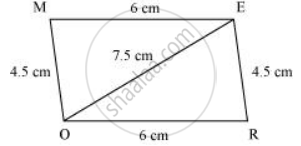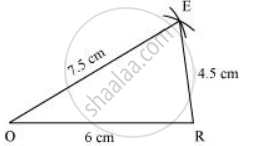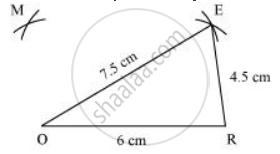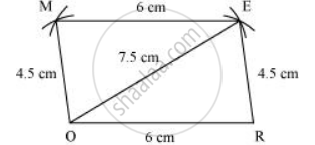Share

# Construct the Following Quadrilaterals. Parallelogram MORE Or = 6 Cm Re = 4.5 Cm Eo = 7.5 Cm - Mathematics

Course
ConceptConstructing a Quadrilateral When the Lengths of Four Sides and a Diagonal Are Given

#### Question

Parallelogram MORE

OR = 6 cm

RE = 4.5 cm

EO = 7.5 cm

#### Solution

We know that opposite sides of a parallelogram are equal in length and also these are parallel to each other.

Hence, ME = OR, MO = ER

A rough sketch of this parallelogram can be drawn as follows.1) Δ EOR can be constructed by using the given measurements as follows.2) Vertex M is 4.5 cm away from vertex O and 6 cm away from vertex E. Therefore, while taking O and E as centres, draw arcs of 4.5 cm radius and 6 cm radius respectively. These will intersect each other at point M.3) Join M to O and E.MORE is the required parallelogram.

Is there an error in this question or solution?

#### APPEARS IN

NCERT Solution for Mathematics Textbook for Class 8 (2018 to Current)
Chapter 4: Practical Geometry
Ex. 4.1 | Q: 1.3 | Page no. 60

#### Video TutorialsVIEW ALL 

Solution Construct the Following Quadrilaterals. Parallelogram MORE Or = 6 Cm Re = 4.5 Cm Eo = 7.5 Cm Concept: Constructing a Quadrilateral - When the Lengths of Four Sides and a Diagonal Are Given.
S